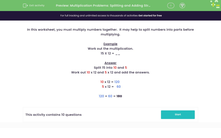# Multiplication Problems: Partition Numbers to Multiple

In this worksheet, students multiply two-digit numbers by splitting one of the numbers and adding the parts if necessary (the Distributive Law).Key stage:  KS 2

Curriculum topic:   Number: Multiplication and Division

Curriculum subtopic:   Solve Multiplication Problems

Difficulty level:#### Worksheet Overview

In this worksheet, we multiply numbers together.  It may help to split numbers into parts before multiplying.

Example

Work out the multiplication.

15 X 12 = __

Split 15 into 10 and 5

Work out 10 x 12 and 5 x 12 and add the answers.

10 x 12 = 120

5 x 12 =   60

120 + 60 = 180

### What is EdPlace?

We're your National Curriculum aligned online education content provider helping each child succeed in English, maths and science from year 1 to GCSE. With an EdPlace account you’ll be able to track and measure progress, helping each child achieve their best. We build confidence and attainment by personalising each child’s learning at a level that suits them.

Get started